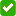### Create an Account

Home / Questions / Yello Bus Lines uses the units-of-activity method in depreciating its buses One bus was pu...

# Yello Bus Lines uses the units-of-activity method in depreciating its buses One bus was purchased on January 1 2015 at a cost of \$236666 Over its 7-year useful life the bus is expected

Yello Bus Lines uses the units-of-activity method in depreciating its buses. One bus was purchased on January 1, 2015, at a cost of \$236,666. Over its 7-year useful life, the bus is expected to be driven 144,400 miles. Salvage value is expected to be \$7,070.

Warning

 Don't show me this message again for the assignment

Ok

Cancel(a)Your answer is correct.
Compute the depreciable cost per unit. (Round answer to 2 decimal places, e.g. 0.50.)
 Depreciation cost per unit \$1.59

Warning

 Don't show me this message again for the assignment

Ok

Cancel

 Attempts: 1 of 2 used(b)

Prepare a depreciation schedule assuming actual mileage was: 2015, 38,100; 2016, 42,200; 2017, 36,200; and 2018, 27,900. (Round cost per unit to 2 decimal places, e.g. 15.25 and all other answers to 0 decimal places, e.g. 5,275.)
 Computation End of Year Year Units ofActivity Af— DepreciationCost/Unit = AnnualDepreciationExpense AccumulatedDepreciation BookValue 2015\$\$\$\$201620172018Warning

 Don't show me this message again for the assignment

Ok

Cancel

Show transcribed image text Yello Bus Lines uses the units-of-activity method in depreciating its buses. One bus was purchased on January 1, 2015, at a cost of \$236,666. Over its 7-year useful life, the bus is expected to be driven 144,400 miles. Salvage value is expected to be \$7,070. Warning Don't show me this message again for the assignment Ok Cancel Warning Don't show me this message again for the assignment Ok Cancel 2018 2017 2016 \$ \$ \$ \$ (b) Prepare a depreciation schedule assuming actual mileage was: 2015, 38,100; 2016, 42,200; 2017, 36,200; and 2018, 27,900. (Round cost per unit to 2 decimal places, e.g. 15.25 and all other answers to 0 decimal places, e.g. 5,275.) Computation End of Year Year Units of Activity A???? Depreciation Cost/Unit = Annual Depreciation Expense Accumulated Depreciation Book Value 2015 1.59 Warning Don't show me this message again for the assignment Ok Cancel Link to Text Attempts: 1 of 2 used Your answer is correct. Compute the depreciable cost per unit. (Round answer to 2 decimal places, e.g. 0.50.) Depreciation cost per unit \$ (a)

Jun 24 2020 View more View LessSubscribe To Get Solution